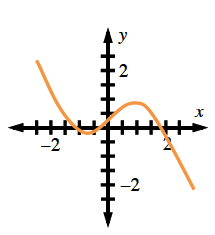### Home > APCALC > Chapter 5 > Lesson 5.1.3 > Problem5-32

5-32.

Examine the integrals below. Consider the multiple tools available for evaluating integrals and use the best strategy for each part. Evaluate each integral and briefly describe your method.

1. $\int _ { 1 } ^ { 4 } ( 2 x + 1 ) ^ { 2 } d x$

Expand the integrand first.

1. $\int _ { - \pi } ^ { \pi } x \operatorname { cos } ( x ) d x$

Can you calculate the integral just by looking at the graph?Without graphing, how could you have recognized that the integrand is odd?

1. $\int ( 2 \operatorname { sin } ^ { 2 } ( x ) + 2 \operatorname { cos } ^ { 2 } ( x ) ) d x$

Factor and use a trigonometry identity.

$\int 2(\text{sin}^{2}x+\text{cos}^{2}x)dx=\int 2dx= 2x+C$

1. $\int ( \frac { t ^ { 2 } } { 2 } - \frac { 2 } { t ^ { 2 } } ) d t$

How could you simplify so you can use the Power Rule?